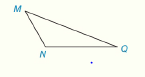Chapter 2.CT, Problem 17CT### Elementary Geometry for College St...

6th Edition
Daniel C. Alexander + 1 other
ISBN: 9781285195698

#### Solutions

Chapter
Section### Elementary Geometry for College St...

6th Edition
Daniel C. Alexander + 1 other
ISBN: 9781285195698
Textbook Problem
1 views

# In Exercise 16 and 18, complete the missing statements or reasons for each proof. Use an indirect proof to completethe following proof. Given: Δ M N Q with m ∠ N = 120 ° Prove: ∠ M and ∠ Q are not complementary.To determine

To prove:

The given statement.

Explanation

For indirect proof, consider M, and Q are complementary. According to the corollary, the sum of two complementary angle will be 90°.

Given:

The following figure shows the given diagram.

Given that, ΔMNQ with mN=120°.

Approach:

Write the sum of complementary angle.

mM+mQ=90°

According to the theorem of triangle, the sum of three angle will be 90°

### Still sussing out bartleby?

Check out a sample textbook solution.

See a sample solution

#### The Solution to Your Study Problems

Bartleby provides explanations to thousands of textbook problems written by our experts, many with advanced degrees!

Get Started

#### In Exercises 516, evaluate the given quantity. log22

Finite Mathematics and Applied Calculus (MindTap Course List)

#### If limx0sinxx=1, the angle x must be measured in: a) radians b) degrees c) it does not matter

Study Guide for Stewart's Single Variable Calculus: Early Transcendentals, 8th

#### Evaluate each sum. k=25(k2+3k)

College Algebra (MindTap Course List)

#### Define the validity of measurement and explain why and how it is measured.

Research Methods for the Behavioral Sciences (MindTap Course List)